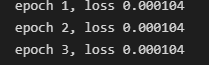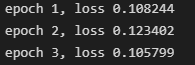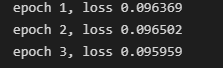# 线性回归的简洁实现

## 练习一

• 将损失函数的计算方式定义为整个损失的和
``````loss = nn.MSELoss(reduction='sum')
trainer = torch.optim.SGD(net.parameters(), lr=0.03)  #指定需要优化的参数 还有学习率
num_epochs = 3
for epoch in range(num_epochs):
for X, y in data_iter:
l = loss(net(X) ,y) #这里的net返回输入x经过定义的网络所计算出的值
l.backward() #损失函数进行反向传播 求参数的梯度
trainer.step()  #trainer步进 根据指定的优化算法进行参数的寻优
l = loss(net(features), labels) #根据上面的参数优化结果计算参数对整个数据集的拟合状态 以loss进行反映
print(f'epoch {epoch + 1}, loss {l:f}')
``````
• 在上述情况下改变学习率
``````trainer = torch.optim.SGD(net.parameters(), lr=0.03/10)  #指定需要优化的参数 还有学习率
num_epochs = 3
for epoch in range(num_epochs):
for X, y in data_iter:
l = loss(net(X), y)  #这里的net返回输入x经过定义的网络所计算出的值
l.backward()  #损失函数进行反向传播 求参数的梯度
trainer.step()  #trainer步进 根据指定的优化算法进行参数的寻优
l = loss(net(features), labels)  #根据上面的参数优化结果计算参数对整个数据集的拟合状态 以loss进行反映
print(f'epoch {epoch + 1}, loss {l:f}')
``````

## 总结

• 以平均值作为损失函数• 以和作为损失函数，不改变学习率• 以和作为损失函数，改变学习率14 Likes

1 Like

1 Like

1 Like

1000个样本按batch_size=10划分，分成了100份，迭代一次样本需要100次sgd优化，我猜这个trainer.step()就是每循环一次执行一个batch_size的sgd优化

1 Like

1 Like

Pytorch是有提供nn.HuberLoss的哦，你再看看

Thanks。What you said is really good

The problem have been posted to python - Pytorch CUDA error: CUBLAS_STATUS_INVALID_VALUE when calling `cublasSgemm( handle, opa, opb, m, n, k, &alpha, a, lda, b, ldb, &beta, c, ldc)` - Stack Overflow
Any suggestion is appreciated!

1. `nn.MSELoss(reduction='sum')`: 当设置`reduction='sum'`时，损失函数将计算预测值与目标值之间的平方差，并对所有平方差求和。这意味着你会得到一个单一值，表示所有样本的总损失。
2. `nn.MSELoss()`（默认`reduction='mean'`）：当没有指定`reduction`参数或将其设置为`'mean'`时，损失函数将计算预测值与目标值之间的平方差，并对所有平方差求平均。这意味着你会得到一个单一值，表示所有样本的平均损失。
实际上我们可以得出：`nn.MSELoss()` = `nn.MSELoss(reduction='sum')`/batch_size
因此，当我们用nn.MSELoss(reduction=‘sum’)替代 `nn.MSELoss()`时，只需要对lr除以batch_size就行。因为在求导过程中，常数项作为系数保持不变，梯度的大小也乘上了batch_size

``````def Huber(pred , true , sigma = 0.005):
error = abs(pred.detach().numpy() - true.detach().numpy())
return torch.tensor(np.where(error < sigma , error - sigma / 2 , 0.5 * sigma * error ** 2) , requires_grad= True).mean()
``````

def huber_loss(y_hat, y, beta = 0.005):
error = torch.abs(y_hat - y.detach())
return torch.where(error < beta , 0.5 * error ** 2 / beta, error - 0.5 * beta)